JMM 2016

# Basic ideas

• Sampling distributions approximated by theoretical distributions
• parameters of these distributions are estimated from data
• p-values and CIs based on formulas & estimated parameters

$t = \frac{ \overline{x} - \mu}{s / \sqrt{n}}$

#### SBI Approach

• Sampling distributions are approximated by simulation
• p-values and CIs are computed from simulated data

• Often used on first day of class

• Story

• woman claims she can tell whether milk has been poured into tea or vice versa.

• Question: How do we test this claim?

• Can replace with any test of a proportion

• fair coin, Dorris and Buzz, multiple-choice guessing, ESP, Hugo, ...

# Computer Simulation

Use rflip() to simulate flipping coins

rflip()
##
## Flipping 1 coin [ Prob(Heads) = 0.5 ] ...
##
## H
##
## Number of Heads: 1 [Proportion Heads: 1]

# Computer Simulation

Faster if we flip multiple coins at once:

rflip(10)
##
## Flipping 10 coins [ Prob(Heads) = 0.5 ] ...
##
## H H H T T T H H H T
##
## Number of Heads: 6 [Proportion Heads: 0.6]
• easier to consider heads = correct; tails = incorrect than to compare with a given pattern
• this switch bothers me more than it bothers my students

# Let's do that a lot of times

rflip(10) simulates 1 lady tasting 10 cups 1 time.

We can do that many times to see how guessing ladies do:

do(2) * rflip(10)
##    n heads tails prop
## 1 10     6     4  0.6
## 2 10     5     5  0.5
• do() is clever about what it remembers
• 2 isn't many -- we'll do many next.

# The distribution of guessing ladies

Ladies <- do(5000) * rflip(10)
head(Ladies, 1)
##    n heads tails prop
## 1 10     4     6  0.4
histogram( ~ heads, data = Ladies, width = 1 )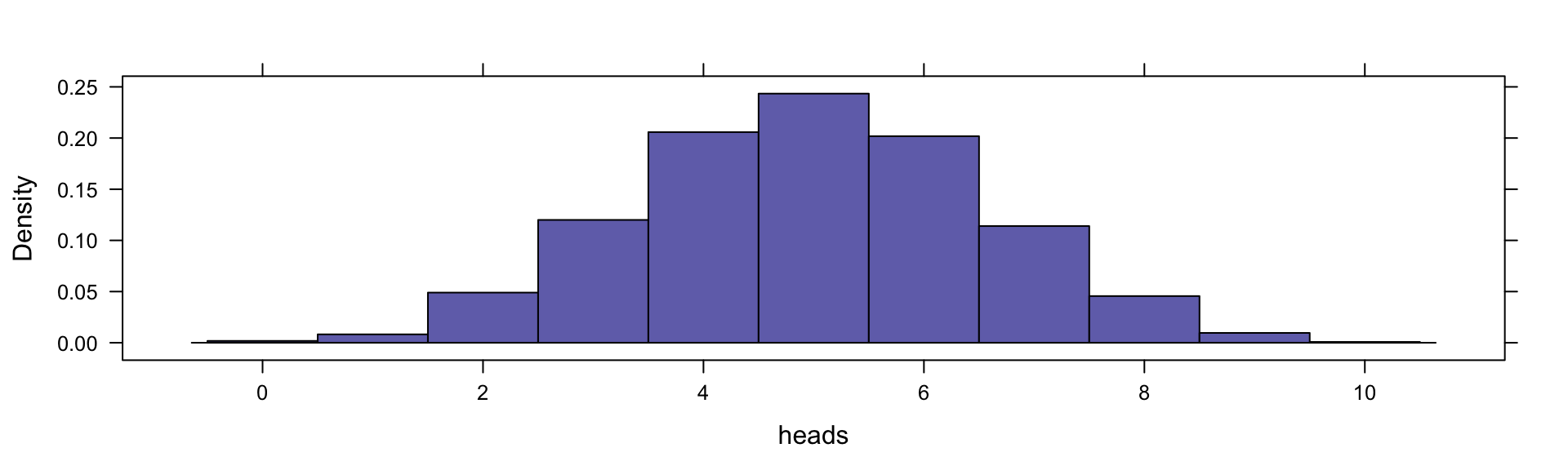# How often does guessing score 9 or 10?

tally( ~(heads >= 9) , data = Ladies)
##
##  TRUE FALSE
##    52  4948

# How often does guessing score 9 or 10?

tally( ~(heads >= 9) , data = Ladies,
format = "prop")
##
##   TRUE  FALSE
## 0.0104 0.9896

# How often does guessing score 9 or 10?

tally( ~(heads >= 9) , data = Ladies,
format = "prop")
##
##   TRUE  FALSE
## 0.0104 0.9896
 prop( ~(heads >= 9), data = Ladies)
##   TRUE
## 0.0104

# A general approach to randomization

### Three Step Program

1. Do it for your data
2. Do it for random data
3. Do it lots of times for random data

definition of it is usually natural

definition of random is important ... but can often be handled by

• shuffle() or
• resample()

# Example: Is there a difference in means?

Data: HELPrct

Question: Do the mean ages of men and women differ?

• Of course, there will be a difference in our data

• Want to know whether that difference could be due merely to randomness

# Example: Do mean ages differ by sex?

diffmean(age ~ sex, data = HELPrct)
##   diffmean
## -0.7841284
bwplot( sex ~ age, data = HELPrct)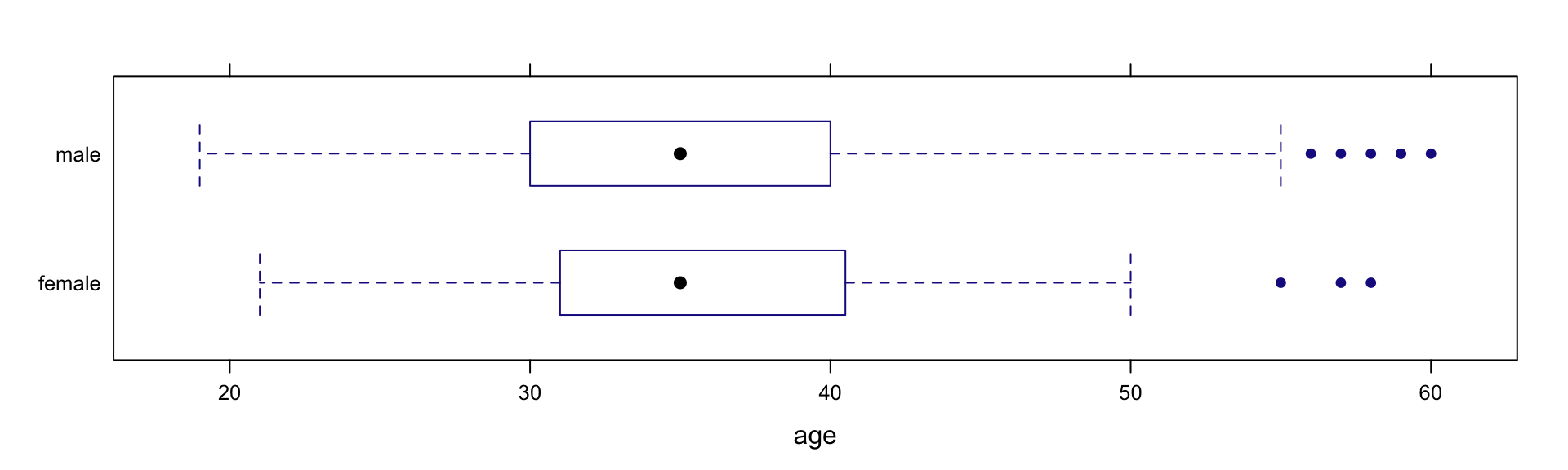# Example: Do mean ages differ by sex?

do(1) *
diffmean(age ~ shuffle(sex), data = HELPrct)
##    diffmean
## 1 0.1090973
Null <- do(5000) *
diffmean(age ~ shuffle(sex), data = HELPrct)

# Example: Do mean ages differ by sex?

2 * prop( ~ (diffmean <= -0.7841), data = Null)
##   TRUE
## 0.3588
histogram( ~ diffmean, data = Null, v = -.7841)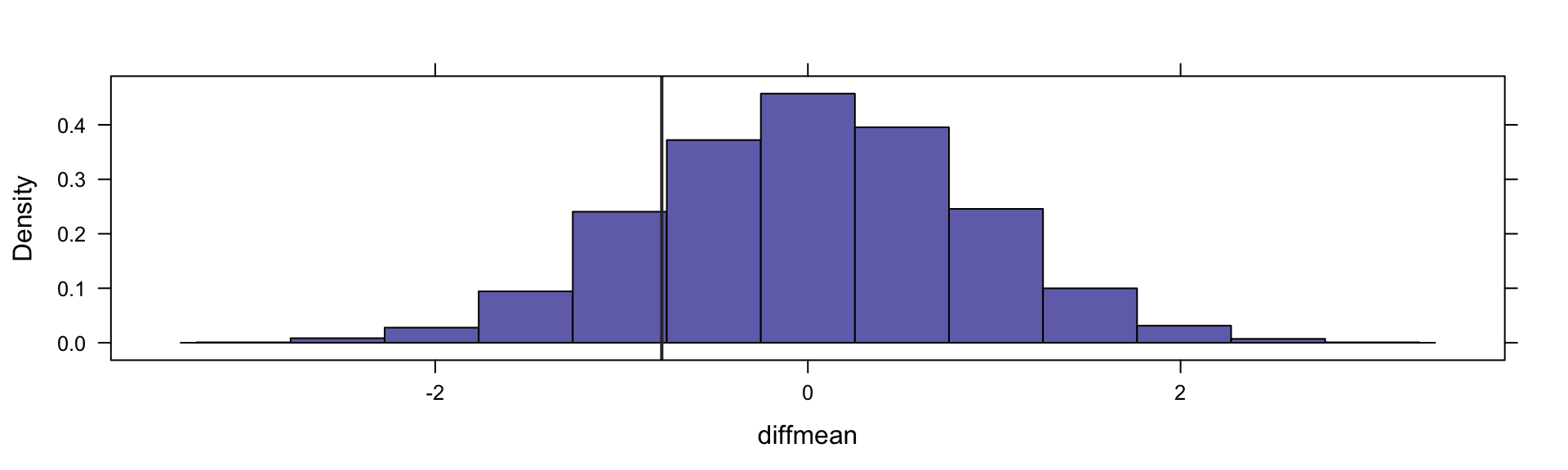# A Little Wrinkle

We should include the observed test statistic in the Null Distribution.

• If the Null Hypothesis is true, the observed test statistic belongs
• Avoids the problem of obtaining a p-value of 0

prop() computes proportions after adding 1 to numerator and denominator.

2 * prop1( ~ (diffmean <= -0.7841), data = Null)
##      TRUE
## 0.3591282

# Example: Bootstrap for difference in means

Bootstrap <- do(5000) *
diffmean(age ~ sex, data= resample(HELPrct))

histogram( ~ diffmean, data = Bootstrap,
v = -.7841, glwd = 4 )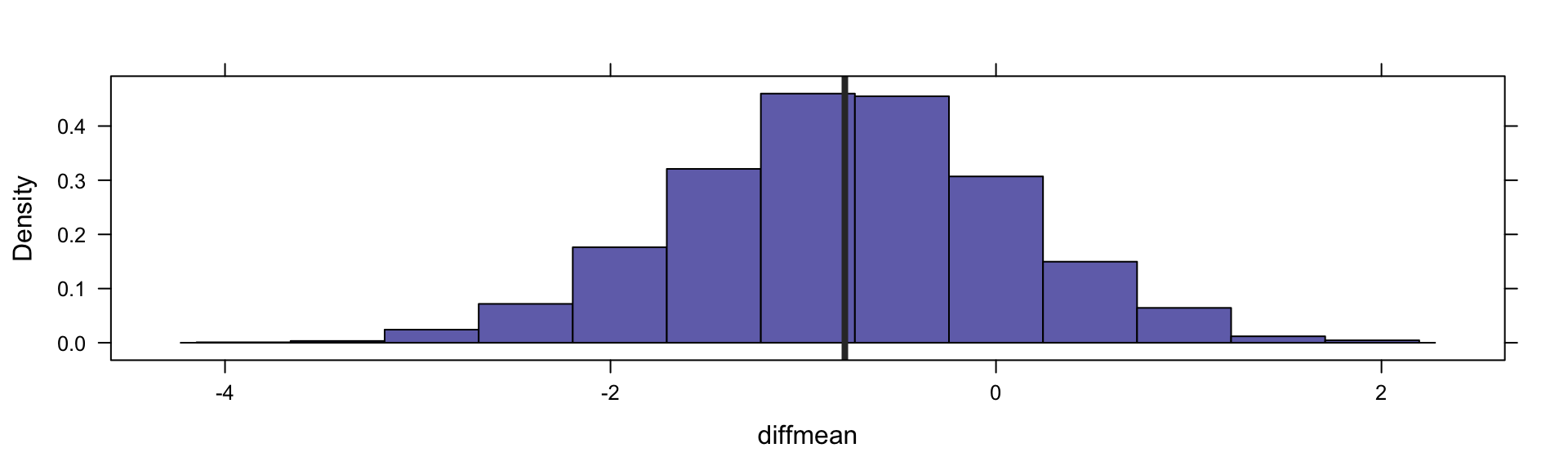# Example: CI for difference in mean ages

autosize: true

cdata( ~ diffmean, data = Bootstrap, p = .95)
##        low         hi  central.p
## -2.4787104  0.8894968  0.9500000
confint(Bootstrap, method = "quantile")
##       name    lower     upper level     method   estimate
## 1 diffmean -2.47871 0.8894968  0.95 percentile -0.7841284

# Example: CI for difference in mean ages

autosize: true

confint(Bootstrap)  # default uses st. err
##       name     lower     upper level method   estimate margin.of.error  df
## 1 diffmean -2.433334 0.8937444  0.95 stderr -0.7841284        1.663539 452

# Randomization and linear models

do(1) * lm(width ~ length, data = KidsFeet)
##   Intercept    length     sigma r.squared        F numdf dendf
## 1  2.862276 0.2479478 0.3963356 0.4110041 25.81878     1    37
do(3) * lm(width ~ shuffle(length), data = KidsFeet)
##   Intercept     length.     sigma   r.squared         F numdf dendf
## 1 11.639314 -0.10706623 0.4962420 0.076635651 3.0708562     1    37
## 2 11.541875 -0.10312500 0.4977279 0.071097404 2.8319481     1    37
## 3  9.754237 -0.03081856 0.5147824 0.006349662 0.2364388     1    37

# Randomization and linear models

do(1) *
lm(width ~ length + sex, data = KidsFeet)
##   Intercept   length       sexG     sigma r.squared        F numdf dendf
## 1  3.641168 0.221025 -0.2325175 0.3848905 0.4595428 15.30513     2    36
do(3) *
lm( width ~ length + shuffle(sex),
data = KidsFeet)
##   Intercept    length       sex.G     sigma r.squared        F numdf dendf
## 1  2.942851 0.2431545  0.07785347 0.3998086 0.4168355 12.86608     2    36
## 2  3.450856 0.2282462 -0.20833605 0.3878261 0.4512672 14.80285     2    36
## 3  2.842685 0.2484316  0.01565872 0.4017205 0.4112447 12.57297     2    36

# Randomization and linear models

Null <- do(5000) *
lm(width ~ length + shuffle(sex), data = KidsFeet)
histogram( ~ sex.G, data = Null,
v = -0.2325, glwd = 4)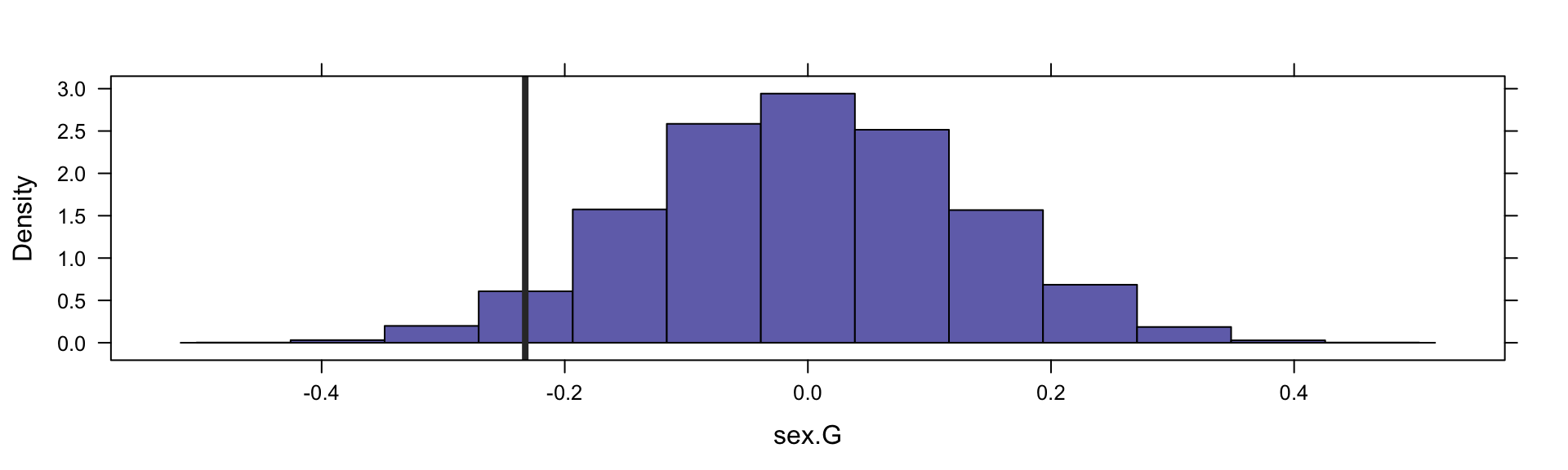# Randomization and linear models

histogram(~ sex.G, data = Null,
v = -0.2325, glwd = 4)prop1(~ (sex.G <= -0.2325), data = Null)
##       TRUE
## 0.03719256`